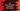# C# array GetLength method explanation with example## C# array GetLength method:

GetLength method is used to find the length of an array in C#. In this post, we will learn how to use GetLength method to find the array length with an example.

### Example of GetLength:

GetLength is defined as below:

``public int GetLength (int dimension);``

Here, dimension is a zero-based dimension of the array.

GetLength returns a 32-bit integer value, i.e. the length of an array. Let’s take a look at the below program that uses GetLength to find the length:

``````using System;

public class Program {

public static void Main(string[] args) {
int[] arr = {4, 3, 2, 1};
int arrLength = arr.GetLength(0);

Console.WriteLine("Array Length : "+arrLength);
}
}``````

It will print 4 as the output.

### Exception:

It throws IndexOutOfRangeException if the dimension is less than zero or dimension is equal or greater than Rank. For one dimensional array, it is 1, for two dimensional array, it is 2 etc.